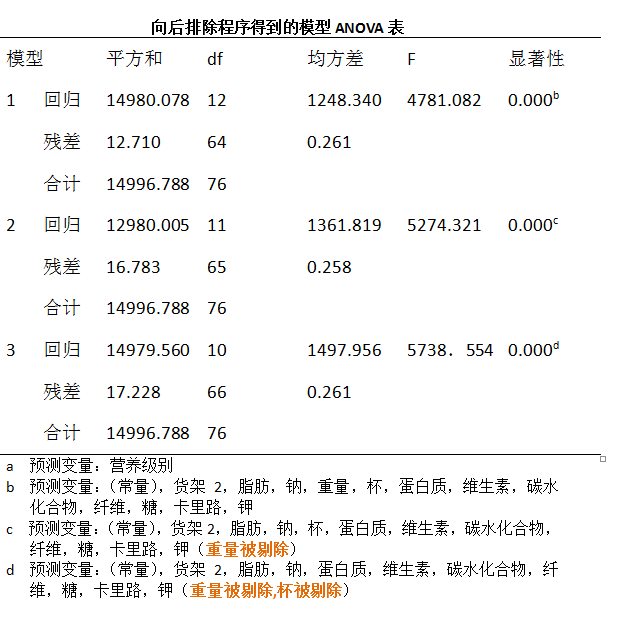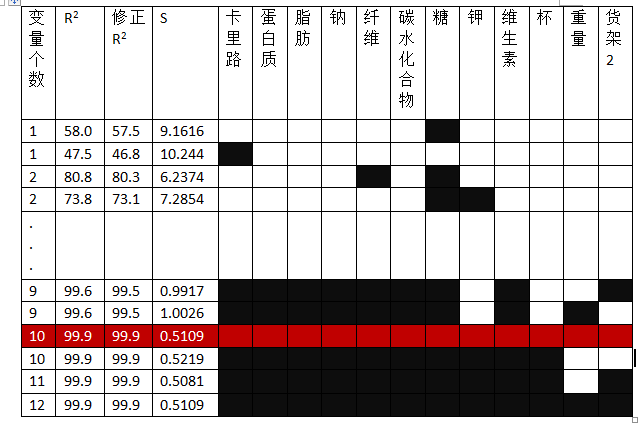# 数据挖掘方法之四：多重共线性及变量选择方法

## 一 概念

### 1分析之前

#### a.逐个计算预测变量之间的相关系数

> cor(sugar$sugars,sugar$shelf)
 0.1004379
> cor(sugar$fiber,sugar$potass)
 0.9033737


#### b.为预测变量建立矩阵图

> #同时画多个变量的对照图需要使用 car包中的 scatterplotMatrix函数
>install.packages("car")
>library(car)
>#使用谷物数据集的 “糖”，“纤维”，“钾”三列数据
> sugar_frame<-as.data.frame(sugar[,c("糖","纤维","钾")])
>#画出对照图


### 1.2 分析之后：方差膨胀因子(variance inflation factors,VIFs)

$VIF_i$ 的变化对第i个系数的变化率Sbi如何产生影响，有如下公式：

• VIF>=5 模型有中度的多重共线性（相当于 $R^2=0.08$ ）
• VIF>=10 模型中有严重多重共线性(相当于 $R^2=0.90$ )

> #回归拟合
> fit<-lm(data=sugar,rating~sugars+fiber+potass)
>#注意，我们只是用了sugar数据集中包含“糖”，“纤维”，“钾”三列数据的sugar_frame
>#进行膨胀因子计算时，需要使用gvlma包中的vif函数，因此需要先安装
> install.packages("gvlma")
> library(gvlma)
Warning message:

>#线性模型的综合验证
> gvlma(fit)
Call:
lm(formula = rating ~ sugars + fiber + potass, data = sugar)

Coefficients:
(Intercept)       sugars        fiber       potass
52.6762      -2.0510       4.3701      -0.0543

ASSESSMENT OF THE LINEAR MODEL ASSUMPTIONS
USING THE GLOBAL TEST ON 4 DEGREES-OF-FREEDOM:
Level of Significance =  0.05

Call:
gvlma(x = fit)

Value p-value                   Decision
Global Stat        7.24415 0.12353    Assumptions acceptable.
Skewness           5.61716 0.01779 Assumptions NOT satisfied!
Kurtsis           0.02125 0.88411    Assumptions acceptable.
Link Function      0.40164 0.52624    Assumptions acceptable.
Heteroscedasticity 1.20410 0.27250    Assumptions acceptable.
>#查看膨胀因子 vif
> vif(fit)
sugars    fiber   potass
1.164237 6.327286 6.204047



## 二. 变量选择方法

• 向前选择
• 向后排除
• 逐步选择
• 最优子集

### 2.1 向前选择程序

1.对于第一个加入模型的变量，选择与回应变量相关度最高的预测变量（假设为 $x_1$ ）如果所有变量对模型都不重要，则停止,否则执行2

1.  对其余的每个变量，F统计序列式 $F(x_2|x_1),F(x_3|x_1),F(x_4|x_1)$ .第二次通过此算法时是, $F(x_3 x_1,x_2),F(x_4 x_1,x_2)$ 。选择具有最大F统计序列的变量
2. 对 2 选择出来的变量，进行F统计序列的显著性检验。如果结果模型没有重大意义，则停止，否咋将从 2 得到的变量加入到模型中，然后返回2

### 2.2 向后排除程序

1. 在全模型中执行向后排除，即使用所有变量的模型。例如，可能全模型中有4个变量 $x_1,x_2,x_3,x_4$
2. 对于当前模型中的每个变量，计算出它的偏F统计量。第一次是：$F(x_1,x_2,x_3,x_4)、F(x_2|x_1,x_3,x_4)、F(x_3|x_1,x_2,x_4)和F(x_4|x_1,x_2,x_3)$ 。选择具有最小偏F统计量的比那辆，其值用 $F_{min}$表示
3. 检验 $F_{min}$ 的显著性。如果 $F_{min}$ 不显著，从模型中删除与Fmin对应的变量，然后返回执行2，如果 $F_{min}$ 显著，停止这个过程。模型1表示包含所有预测变量，模型2中剔除了重量之外所有预测变量，于是有：

F统计量的值0.28 落在 $F_{1,n-p-2}=F_{1,72}$ 分布的40%点处，对应的p值是0.60，因而重量不应该包含在模型中。

### 2.4 最优子集程序

1. 分析人员需要指定需要多少个（假设为m）供筛选的模型，以及在一个模型中含有最大预测变量个数（假设为n）
2. 对于含有一个预测变量的所有模型，例如：$y=b_0+b_1(糖),y=b_0+b_1(纤维)$,….等。计算对应的 $R^2$ ,修正 $R^2$ 和S值都计算出来，最优的m个模型是基于这些统计值得到。
3. 对于含有两个最优的m个模型是基于这些统计值得到。
4. 重复以上，直到达到最大的预测变量（n）个数，然后分析人员把预测变量个数为1,2,,..n的最优模型罗列，以选择最佳总体模型

[注意，整个过程比下图要复杂，例如变量数为1时，本应该有12行结果，下图中只简要用了两行，其他的也是]图中，每一行代表一个不同的模型，某模型中包含了哪个变量，该变量对应的方格被涂成黑色。如，第一个模型（第一行）仅包含了变量糖；第四个模型（第四行）包含了糖和钾。其中的最优模型子集被红色覆盖的那个模型（也即那一行）。